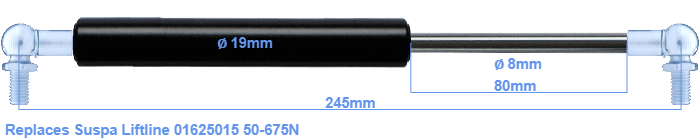# Replacement for Suspa Liftline 01625015 50-675N

\$35.06

Replacement gas spring for the Suspa Liftline 01625015 50-675 Newton. This fits a (possibly already present) ball with a diameter of 10mm. A corresponding ball is included. Brand: Stabilus Industry Line.
 Force Choose an option50 Newton60 Newton80 Newton100 Newton120 Newton140 Newton150 Newton160 Newton180 Newton200 Newton220 Newton240 Newton250 Newton260 Newton280 Newton300 Newton320 Newton340 Newton350 Newton360 Newton380 Newton400 Newton420 Newton440 Newton450 Newton460 Newton480 Newton500 Newton520 Newton540 Newton550 Newton560 Newton580 Newton600 Newton620 Newton640 Newton650 Newton660 Newton675 NewtonClear
This gas spring is also known as 16-2 016 25015, 16-2-121-85-A246-B246.The diameter of the cylinder of this gas spring is 19 millimeters. The diameter of the rod totals 8 mm. The stroke of the gas spring is 80 millimeter. The total length of this replacement gas spring is 245 mm. Keep in mind that this is the length between the centers of the mounting parts. The distance from thread to thread (without mounting parts) is 205 mm. This replacement gas spring has a force of 50-675 Newton. This is not an original gas spring produced by Suspa Liftline, but it can replace a Suspa Liftline gas spring. This is a Stabilus Industry Line gas spring. Nevertheless, the force and dimensions equal.
Category: